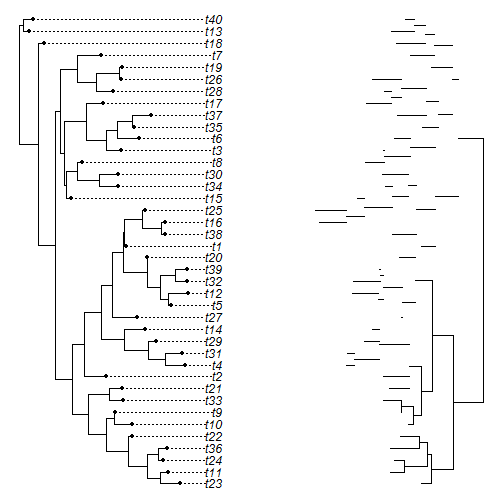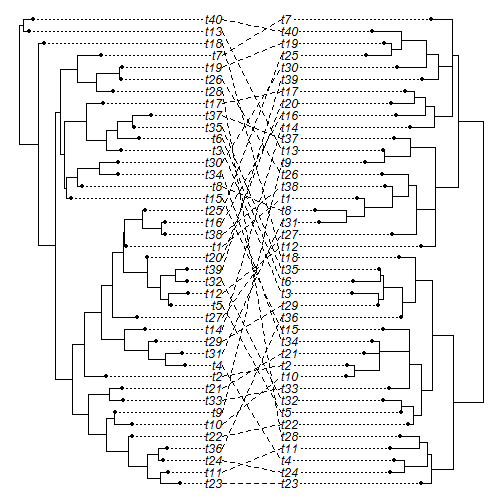## Monday, August 10, 2015

### Bug fix for co-phylogenetic tree plotting when one or both trees contain polytomies

Yesterday, a phytools user & phytools blog reader commented the following about the phytools function for co-phylogenetic plotting, `cophylo` (e.g., 1, 2):

I've been looking for a way to compare two trees while minimizing overlap, so I was glad to see this! I successfully used the cophylo function with two non-ultrametric trees, but when plotting it continues to plot only the horizontal branches of the left tree and then give me this:

Error in xy.coords(x, y) : 'x' and 'y' lengths differ

The two trees are made from the same strains so they are identical in tip labels and number of tips (84 tips, 82 nodes). I'm not really sure why it's giving me the error, any idea what might be causing it?

My one idea is that it is because one of the two trees has polytomies. I can tell this because the number of nodes (82) is fewer than one less than the number of tips (84). In fact, simulating trees with polytomies does in fact duplicate the error exactly.

So, for instance:

``````library(phytools)
t1<-rtree(n=40)
t2<-rtree(n=40)
ii<-which(t2\$edge[,2]>Ntip(t2))
t2\$edge.length[sample(ii,4)]<-0
t2<-di2multi(t2)
obj<-cophylo(t1,t2)
``````
``````## Rotating nodes to optimize matching...
## Done.
``````
``````plot(obj)
``````
``````## Error in xy.coords(x, y): 'x' and 'y' lengths differ
``````The simplest work-around is to simply resolve the polytomous tree, in this case `t2`, randomly using branches of zero length:

``````obj<-cophylo(t1,multi2di(t2))
``````
``````## Rotating nodes to optimize matching...
## Done.
``````
``````plot(obj)
``````Though this works, of course, it does not allow, for example, descendant edges to be spaced properly from a polytomous node.

The solution, however, was super simple. All that is required was that I change:

```## plot vertical relationships
for(i in 1:tree\$Nnode+n) lines(d*X[which(cw\$edge[,1]==i),1],
range(y[cw\$edge[which(cw\$edge[,1]==i),2]]),lwd=lwd,lend=2)
```

to:

```## plot vertical relationships
for(i in 1:tree\$Nnode+n) lines(d*X[which(cw\$edge[,1]==i),1],
sort(y[cw\$edge[which(cw\$edge[,1]==i),2]]),lwd=lwd,lend=2)
```

This is because when there is more than one edge descending from `cw\$edge[,1]==i` (our current node), then `X[which(cw\$edge[,1]==i),1]` is a vector containing the x containing a number of elements equal to the number of descendants, whereas `range(y[cw\$edge[which(cw\$edge[,1]==i),2]])` can only contain two values: the range, which are the vertical start & end points of the vertical lines we want to draw. By changing it to: `sort(y[cw\$edge[which(cw\$edge[,1]==i),2]])` I now just have the y-coordinates, in a line, of all edges arising from that node.

The modified code is here and it will be in the next version of phytools.

``````load("http://www.phytools.org/cophylo/v0.6/cophylo.R")
``````
``````## Warning in readChar(con, 5L, useBytes = TRUE): cannot open compressed file
## 'http://www.phytools.org/cophylo/v0.6/cophylo.R', probable reason 'Invalid
## argument'
``````
``````## Error in readChar(con, 5L, useBytes = TRUE): cannot open the connection
``````
``````obj<-cophylo(tr1,tr2)
``````
``````## Error in inherits(tr1, "phylo"): object 'tr1' not found
``````
``````plot(obj)
``````It works!

1.Worked perfectly this time, thanks for the help!
Rachel

2.Hi Liam,

This is excellent. One quick questions because my R skills are admittedly weak - is there a way to maintain support values in the co-plotted trees? I don't see any easy way to do this in the source for cophylo.R.

1.This might address this question: http://blog.phytools.org/2015/10/node-edge-tip-labels-for-plotted.html.

Let me know. Liam

Note: due to the very large amount of spam, all comments are now automatically submitted for moderation.